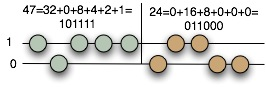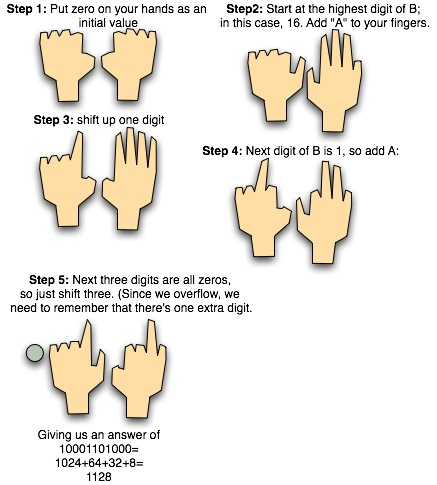# Who needs a calculator? Multiplying with Your Fingers

To do multiplication with your fingers in binary is very easy: it’s just a mixture of addition and bit-shifting. The only real trick is memory: to multiply a×b, you need to remember the binary digits of both x and y, which can be a bit of a trick for 10 digit binary numbers.
The trick that I like is to use coins. Lay out a bunch of coins: one for each binary digit of a, and one for each binary digit of b. Put two lines on a piece of paper: one line will be the ones, the other will be the zeros. So, for example, to multiple 47 times 24, you’d start with the following:The coins on the paper are your guide, to help you remember the two numbers you’re multiplying. Now the basic algorithm. You start with zero on your fingers; then, starting with the *largest* digit of *B*:
1. Take the current sum on your hands, and multiply it by two. Multiplying by
two is just a simple shift operation – shift each digit up to the next highest
position.
2. If the current digit is “1”, then add “A” to what’s on your fingers.
3. Move to the next digit of “B”.
So, for example:That’s all there is to it. It’s really easy; once you’ve
to multiplication this way is very straightforward and
mechanical.
For the particularly clever folks out there, you’ll notice that this is
pretty much the same algorithm that we used for [multiplying roman numerals](http://scienceblogs.com/goodmath/2006/08/roman_numerals_and_arithmetic.php).

## 0 thoughts on “Who needs a calculator? Multiplying with Your Fingers”

1.Drekab

I think your coin diagram is off, you need a sixth coin. Otherwise its a pretty neat trick.

2.Drekab

Sorry, I’m still thoroughly confused, maybe if you put the total value after each step for us slower readers? And how are you getting one as a digit of B? I thought B was 24. I’ll keep trying but this seems to be going over my head.

3.Xanthir, FCD

Drekab: Let me run through it – I also found this one to be a little bit confusing.
Okay, first, just to help keep the numbers in one’s head, Mark recommends displaying them with coins. This is helpful.
Now, here’s how you go. First, bitshift the value in your hand. Since it’s 0 right now, that means don’t do anything.
Second, check the largest digit of B (written in binary). Is it a 1? If so, add A to what you have on your hand. Discard the largest digit of B and repeat from the first step.
So in this case (47 x 24, or 101111 x 11000), we start by clearing our fingers (a closed fist). Then, we look at the first digit of B (a 1) and load A (47) into our fingers. So we now have all the fingers of our right hand up except for the pinky, and the thumb of the left hand up. We discard the largest digit of B, leaving us with 1000.
Now, bitshift your fingers. This gives us the right thumb down and all right fingers up, the left thumb down and the right pointer up. Now, check the digit of B that we’re looking at. It’s a 1, so we add A to our fingers again. We’re now at 10001101 on our fingers (thumb, middle, and ring up on right hand, middle up on left hand), if I’m working it correctly. Discard the current digit of B.
Bitshift your fingers again (pointer, ring, pinky on right, ring on left). What’s the current value of B? 0, so we don’t add anything. Discard that 0.
Bitshift twice more without adding, as B has nothing but zeros. We’ve exhausted B, and so we’re done. Final value on our hand is ring on right, thumb, pointer, and mysterious 6th finger on left. That translates to 100 0110 1000 in binary, or 468 in Hex, or 1024+96+8=1128 in Decimal. Calculator gives 1128 as well, so we’re correct.
The complicated part is adding – once you get fast with that, this stuff is easy.

4.David Harmon

Personally, I do not find “bitshifting my fingers” to be a natural operation! Pretty awkward, in fact. Especially when the ring and middle fingers get involved.

5.Mark C. Chu-Carroll

David:
It’s just a matter of practice. It *can* be very natural. The main trick is that you don’t try to do it all at once; you do it one bit at a time. You start with the finger that’s the highest-order bit, and make it the same as the finger next to it, and then just keep on moving through your fingers.

6.Mustafa Mond, FCD

Let me give it a try…
Scissors cut paper. I win!

7.battlepanda

I think I’m going to need a definition of “bitshifting” here…also, there is supposed to be six coins in each row, right?

8.Xanthir, FCD

Yeah, Mark left off the last coin in each row. ^_^
Bitshifting is, well, shifting the bits. It simply means moving all the bits to the left or the right. In binary, multiplying by two is the same as bitshifting to the left. For example:
If I write, say, 13 in binary, I get 1101. If I bitshift it to the left, I get 11010. This is 26, so you see that bitshifting to the left multiplied by 2!
Bitshifting to the right is the same as dividing by two. 1101 right-shifted is 110, or 6, which is half of 13.

9.Xanthir, FCD

To be thorough, 1101 bitshifted to the right is 110.1, or 6.5. But usually we’re interested only in the integral portion of non-base10 numbers.

10.Drekab

Xanthir:
Thanks, that helped a lot. I have to admit that I gave up on my fingers and grabbed some more coins, but at least I figured out the principal.

11.Xanthir, FCD

Hey, no problem. Actually, I messed up at one point (I was trying to do bitshifts in the wrong direction, so it was really difficult), and I had to work it out on paper to get back to where I needed to be. There’s no shame in that. ^_^ With practice, though, it should come rather naturally, which is the point.

12.Kadin

Xanthir: regarding the directions you gave, why, after ‘loading’ 47 into your fingers the first time, does the right pinky remain down, and all other right fingers and left thumb rise. Shouldn’t all right fingers except for the thumb rise, and the left thumb, assuming both palms are facing downwards like in the diagram?

13.dude meister

Kadin – the diagram above is wrong, the way they’re discussing things it sounds like the left hand is palm down, right hand palm up.
Instead of lining up coins for the “A” and “B”, use 11 coins in place of your fingers. Makes shifting real easy.